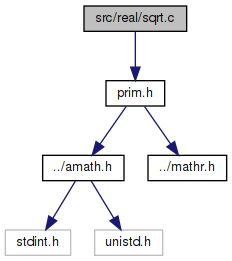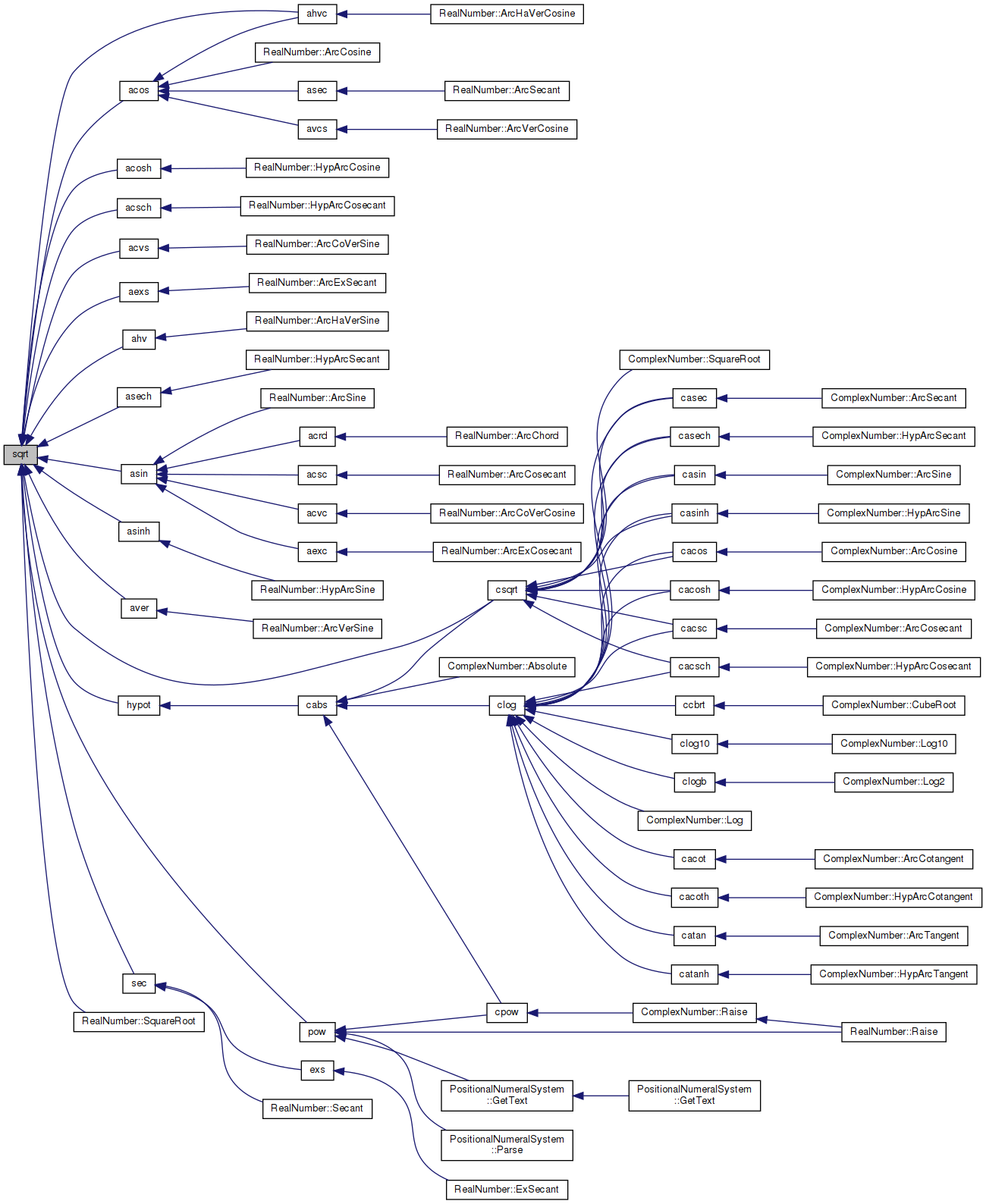amath  1.8.5 Simple command line calculator
sqrt.c File Reference

Square root function. More...

`#include "prim.h"`
Include dependency graph for sqrt.c:Go to the source code of this file.

## Functions

double sqrt (double x)
Square root function. More...

## Variables

static const double one = 1.0

static const double tiny = 1.0e-300

## Detailed Description

Square root function.

Definition in file sqrt.c.

## ◆ sqrt()

 double sqrt ( double x )

Square root function.

Returns
Correctly rounded sqrt
```Method:
Bit by bit method using integer arithmetic. (Slow, but portable)
1. Normalization
Scale x to y in [1,4) with even powers of 2:
find an integer k such that  1 <= (y=x*2^(2k)) < 4, then
sqrt(x) = 2^k * sqrt(y)
2. Bit by bit computation
Let q  = sqrt(y) truncated to i bit after binary point (q = 1),
i                          0
i+1         2
s  = 2*q , and y  =  2   * ( y - q  ).     (1)
i      i            i                 i```
```     To compute q    from q , one checks whether
i+1       i
-(i+1) 2

(q + 2      ) <= y.         (2)
i
-(i+1)
If (2) is false, then q   = q ; otherwise q   = q  + 2      .
i+1   i             i+1   i```
```     With some algebric manipulation, it is not difficult to see
that (2) is equivalent to
-(i+1)
s  +  2       <= y          (3)
i                i```
```     The advantage of (3) is that s  and y  can be computed by
i      i
the following recurrence formula:
if (3) is false```
```         s     =  s  ,  y    = y   ;            (4)
i+1      i         i+1    i```
```         otherwise,
-i                     -(i+1)
s    =  s  + 2  ,  y    = y  -  s  - 2         (5)
i+1      i          i+1    i     i```
```     One may easily use induction to prove (4) and (5).
Note. Since the left hand side of (3) contain only i+2 bits,
it does not necessary to do a full (53-bit) comparison
in (3).
3. Final rounding
After generating the 53 bits result, we compute one more bit.
Together with the remainder, we can decide whether the
result is exact, bigger than 1/2ulp, or less than 1/2ulp
(it will never equal to 1/2ulp).
The rounding mode can be detected by checking whether
huge + tiny is equal to huge, and whether huge - tiny is
equal to huge for some floating point number "huge" and "tiny".```
```Special cases:
sqrt(+-0) = +-0    ... exact
sqrt(inf) = inf
sqrt(-ve) = NaN    ... with invalid signal
sqrt(NaN) = NaN    ... with invalid signal for signaling NaN
```

Definition at line 119 of file sqrt.c.

References one, and tiny.

Referenced by acos(), acosh(), acsch(), acvs(), aexs(), ahv(), ahvc(), asech(), asin(), asinh(), aver(), csqrt(), hypot(), pow(), sec(), and RealNumber::SquareRoot().

120 {
121  double z;
122  int32_t sign = (int32_t)0x80000000;
123  uint32_t r, t1, s1, ix1, q1;
124  int32_t ix0, s0, q, m, t, i;
125
126  EXTRACT_WORDS(ix0, ix1, x);
127
128  // take care of Inf and NaN
129  if ((ix0 & 0x7FF00000) == 0x7FF00000)
130  {
131  // sqrt(NaN)=NaN
132  // sqrt(+inf)=+inf
133  // sqrt(-inf)=sNaN
134  return x * x + x;
135  }
136
137  // take care of zero
138  if (ix0 <= 0)
139  {
140  if (((ix0 & (~sign)) | ix1) == 0)
141  {
142  // sqrt(+-0) = +-0
143  return x;
144  }
145  else if (ix0 < 0)
146  {
147  //sqrt(-ve) = NaN
148  return NAN;
149  }
150  }
151
152  // normalize x
153  m = (ix0 >> 20);
154  if (m == 0)
155  {
156  // subnormal x
157  while (ix0 == 0)
158  {
159  m -= 21;
160  ix0 |= (ix1 >> 11);
161  ix1 <<= 21;
162  }
163
164  for (i = 0; (ix0 & 0x00100000) == 0; i++)
165  {
166  ix0 <<= 1;
167  }
168
169  m -= i - 1;
170  ix0 |= (ix1 >> (32 - i));
171  ix1 <<= i;
172  }
173
174  m -= 1023; // unbias exponent
175  ix0 = (ix0 & 0x000FFFFF) | 0x00100000;
176  if (m & 1)
177  {
178  // odd m, double x to make it even
179  ix0 += ix0 + ((ix1 & sign) >> 31);
180  ix1 += ix1;
181  }
182
183  // m = [m/2]
184  m >>= 1;
185
186  // generate sqrt(x) bit by bit
187  ix0 += ix0 + ((ix1 & sign) >> 31);
188  ix1 += ix1;
189  q = q1 = s0 = s1 = 0; // [q,q1] = sqrt(x)
190  r = 0x00200000; // r = moving bit from right to left
191
192  while (r != 0)
193  {
194  t = s0 + r;
195  if (t <= ix0)
196  {
197  s0 = t + r;
198  ix0 -= t;
199  q += r;
200  }
201  ix0 += ix0 + ((ix1 & sign) >> 31);
202  ix1 += ix1;
203  r >>= 1;
204  }
205
206  r = sign;
207  while (r != 0)
208  {
209  t1 = s1 + r;
210  t = s0;
211  if ((t < ix0) || ((t == ix0) && (t1 <= ix1)))
212  {
213  s1 = t1 + r;
214  if (((t1 & sign) == (uint32_t)sign) && (s1 & sign) == 0)
215  {
216  s0 += 1;
217  }
218  ix0 -= t;
219  if (ix1 < t1)
220  {
221  ix0 -= 1;
222  }
223  ix1 -= t1;
224  q1 += r;
225  }
226
227  ix0 += ix0 + ((ix1 & sign) >> 31);
228  ix1 += ix1;
229  r >>= 1;
230  }
231
232  // use floating add to find out rounding direction
233  if ((ix0 | ix1) != 0)
234  {
235  // trigger inexact flag
236  z = one - tiny;
237  if (z >= one)
238  {
239  z = one + tiny;
240  if (q1 == (uint32_t)0xFFFFFFFF)
241  {
242  q1 = 0;
243  q += 1;
244  }
245  else if (z > one)
246  {
247  if (q1 == (uint32_t)0xFFFFFFFE)
248  {
249  q += 1;
250  }
251  q1 += 2;
252  }
253  else
254  {
255  q1 += (q1 & 1);
256  }
257  }
258  }
259
260  ix0 = (q >> 1) + 0x3FE00000;
261  ix1 = q1 >> 1;
262  if ((q & 1) == 1)
263  {
264  ix1 |= sign;
265  }
266
267  ix0 += (m << 20);
268  INSERT_WORDS(z, ix0, ix1);
269
270  return z;
271 }
#define INSERT_WORDS(d, ix0, ix1)
Set a double from two 32 bit ints.
Definition: prim.h:187
static const double tiny
Definition: sqrt.c:50
#define EXTRACT_WORDS(ix0, ix1, d)
Get two 32 bit ints from a double.
Definition: prim.h:156
static const double one
Definition: sqrt.c:49
#define NAN
Definition: mathr.h:53
Here is the caller graph for this function:## ◆ one

 const double one = 1.0
static

Definition at line 49 of file sqrt.c.

Referenced by sqrt().

## ◆ tiny

 const double tiny = 1.0e-300
static

Definition at line 50 of file sqrt.c.

Referenced by sqrt().